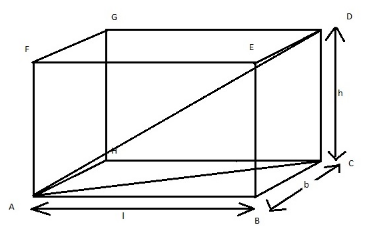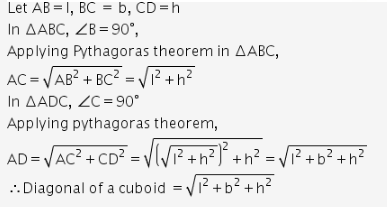# How to prove formula Diagonal of a cuboid = l^2+b^2+h^2

How to prove formula
Diagonal of a cuboid = l2+b2+h2